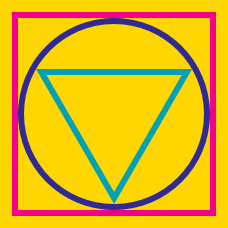Geometry

# Inscribed and Circumscribed Figures: Level 5 Challenges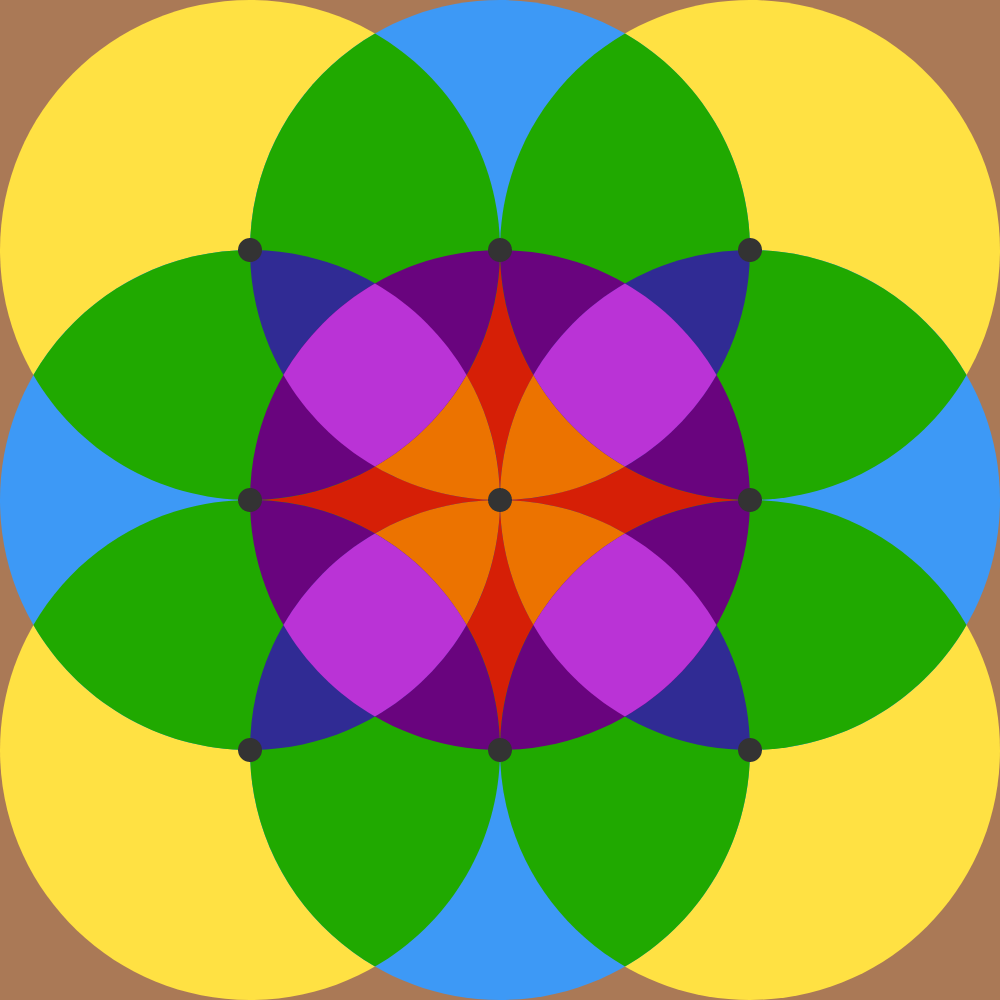Find the area of the brown colored region (outer region) if the radius of each circle is 4 units. Give your answer to 5 decimal places.

Suppose $ABCD$ is a cyclic quadrilateral with side $AD$ being a diameter of length $d$, sides $AB$ and $BC$ both having length $a$ and side $CD$ having length $b$ such that $a,b,d$ are all positive integers with $a \ne b.$

Determine the minimum possible perimeter of $ABCD.$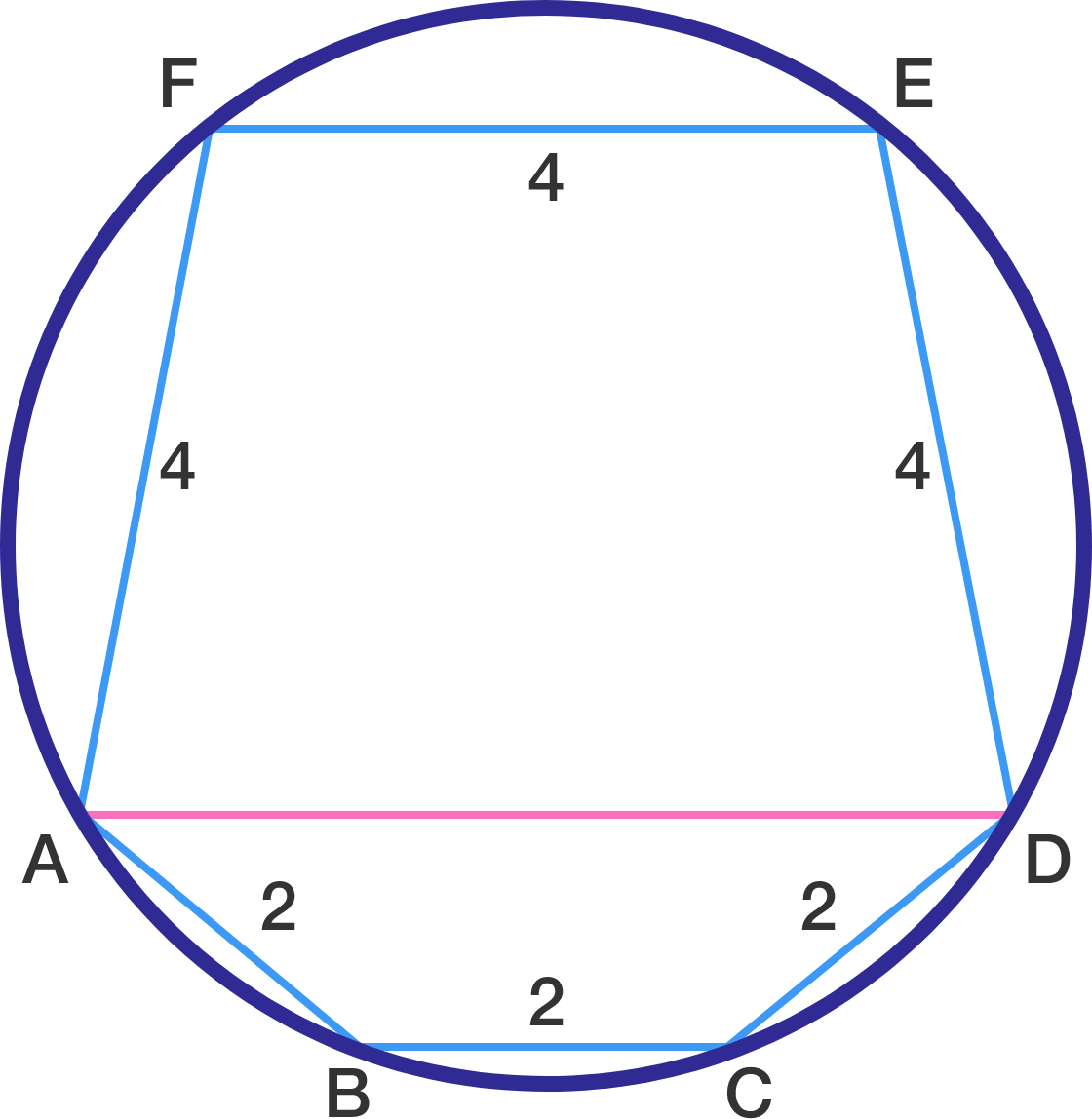An inscribed Hexagon has sides $AF = FE = ED = 4$, $AB = BC = CD = 2$. Furthermore, $ADEF$ and $ADCB$ are trapeziums.

If the length of chord $AD$ is $\frac{ m}{n}$, where $m$ and $n$ are relatively prime integers, find $m + n$.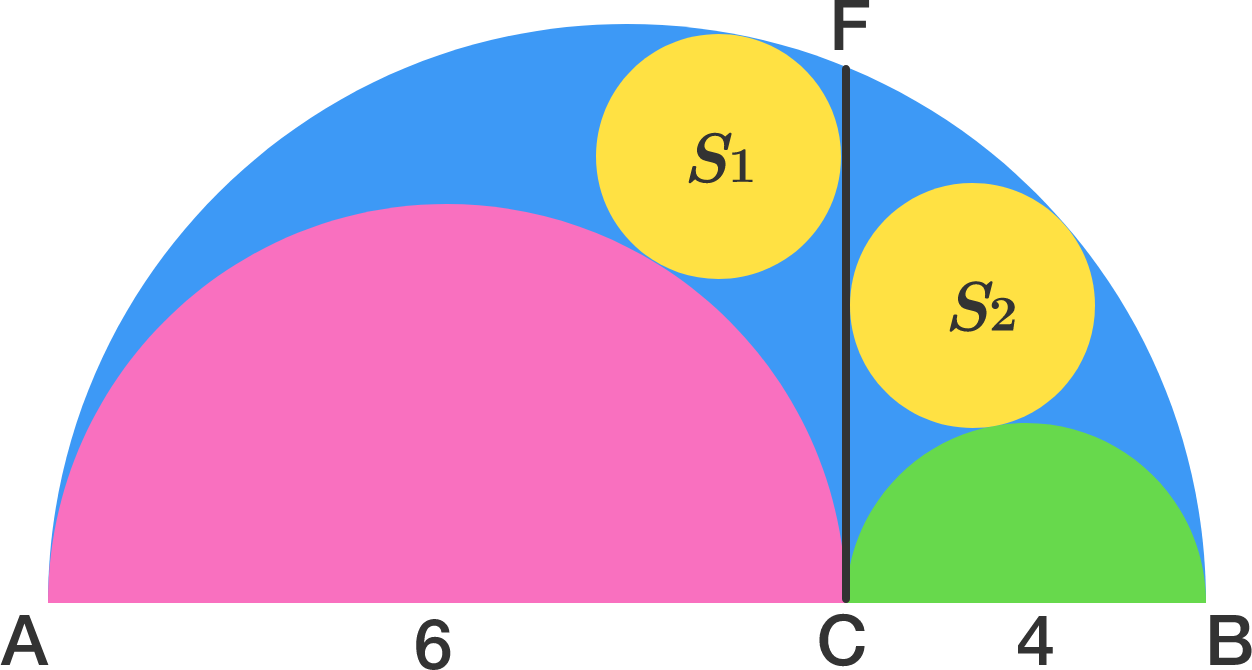In the figure there are three semicircles of diameters $AB$, $AC$, $BC$ and two circles $S_{1}$ and $S_{2}$ which are touching to the line $CF$ ( $CF$ is perpendicular to $AB$ ). Both circles have same radius and are called Archimedean Twins. Now if $AB = 10$, $AC = 6$ and $BC = 4$ then find the radius of circle $S_{1}$.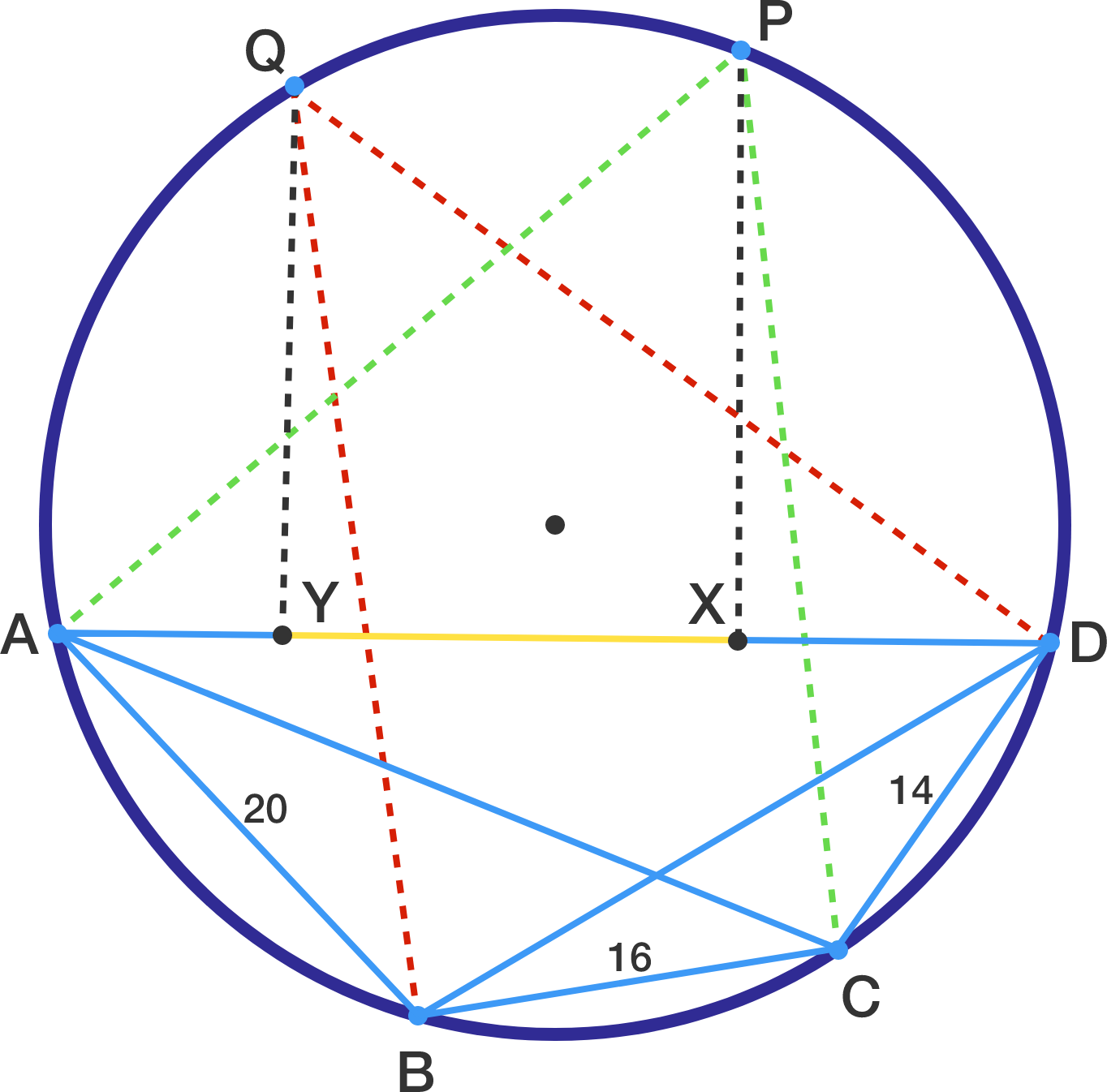Let $ABCD$ be a cyclic quadrilateral with $\angle ABD = \angle ACD >90^{\circ}$.

Let $P$ and $Q$ be the points on major arc $AD$ satisfying $PA=PC$ and $QB=QD$.

Furthermore, let $X$ and $Y$ be the feet of the perpendiculars from $P$ and $Q$ to line $AD$.

If $AB=20$, $CD=14$, and $BC=16$, then find the length of $XY$.

×